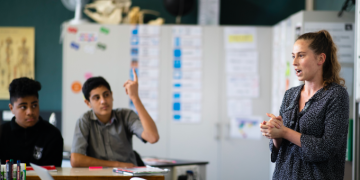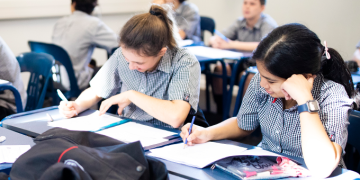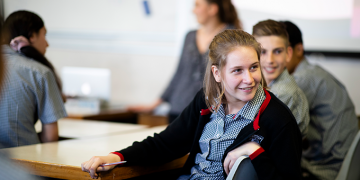##### Teacher hub

Customise your financial capability learning programme here.##### Resources

Explore our wide range of resources to build financial capability knowledge and skills.##### Student hub

Start your journey to become financially capable here.Sorted in Schools is a free financial capability programme fully aligned to the National Curriculum.

# Maths resources

###### This resource encourages students to apply their maths skills and knowledge within the context of financial sustainability. Students explore investments/whakangao, Insurance/inihua, KiwiSaver and retirement/whakatā.

Exploring financial sustainability through maths and statistics is an integrated, cross-curricular resource and can be used in multiple ways. Related resources are available for health and social sciences.

There are a range of resources to choose from, so schools and teachers can design programmes that allow:

• Students to work at their own pace using a student planner
• Teachers to design a programme that suits department, faculty or whole-school planning over a few weeks or a term. This will vary from school to school.

### Mathematics and statistics achievement objectives

In a range of meaningful contexts, students will be engaged in thinking mathematically and statistically. They will solve problems and model situations that require them to:

##### Number and Algebra, Level Four
• Understand addition and subtraction of fractions, decimals and integers
• Find fractions, decimals and percentages of amounts expressed as whole numbers, simple fractions and decimals
• Apply simple linear proportions, including ordering fractions
• Know the equivalent decimal and percentage forms for everyday fractions
• Know the relative size and place-value structure of positive and negative integers, and decimals to three places
• Form and solve simple linear equations
##### Number and Algebra, Level Five
• Reason with linear proportions
• Understand operations on fractions, decimals, percentages and integers
• Use rates and ratios
• Know commonly used fraction, decimal and percentage conversions
• Know and apply standard form, significant figures, rounding and decimal-place value
• Form and solve linear and simple quadratic equations
##### Geometry and Measurement, Level Four
• Convert between metric units, using whole numbers and commonly used decimals
• Use side or edge lengths to find the perimeters and areas of rectangles, parallelograms and triangles, and the volumes of cuboids.
##### Geometry and Measurement, Level Five
• Select and use appropriate metric units for length, area, volume and capacity, weight (mass), temperature, angle and time, with the awareness that measurements are approximate
• Convert between metric units using decimals
• Deduce and use formulae to find the perimeters and areas of polygons and the volumes of prisms
• Find the perimeters and areas of circles and composite shapes and the volumes of prisms, including cylindersEquipping young people for their financial future, embedding good money habits early on.

Hāpaitia te ara tika, pūmau ai te rangatiratanga mo ngā uri whakatipu.

## Māori Medium Education

###### Written in te reo Māori with resources aligned to Te Marautanga o Te Aho Matua and Te Marautanga o Aotearoa.

Hit enter to search or ESC to close